# 【数学建模】六：MATLAB优化模型求解方法(2)：全局优化

离散型问题是建模竞赛中的主流题型，如果判断所研究的问题是组合优化问题， 那么就大概率需要全局优化算法了。历年赛题中， 比较经典的这类问题有灾情巡视、公交车调度、彩票问题、露天矿卡车调度、交巡警服务平台、太阳影子定位等等。可见全局优化问题的求解算法在数学建模中的重要性，这一讲重要就介绍 MATLAB 全局优化技术及相关实例。

1. MATLAB 全局优化概况

MATLAB 中有个全局优化工具箱 ( Global Optimization Toolbox ) ，该工具箱集成了几个主流的全局优化算法，包含全局搜索、多初始点、模式搜索、遗传算法、多目标遗传算法、模拟退火求解器和粒子群求解器， 如图 1 所示。对于目标函数或约束函数连续、不连续、随机、导数不存在以及包含仿真或黑箱函数的优化问题，都可使用这些求解器来求解。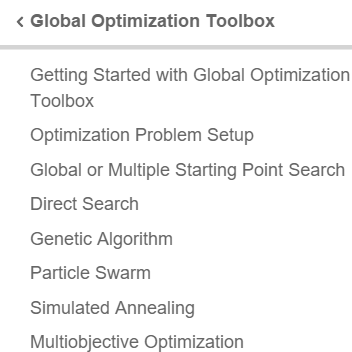2. 遗传算法

• 旅行商问题的数据

plot(x,y,‘Color’,‘red’); hold on;
cities = 40;
locations = zeros(cities,2);
n = 1;
while (n <= cities)
xp = rand*1.5;
yp = rand;
if inpolygon(xp,yp,xx,yy)
locations(n,1) = xp;
locations(n,2) = yp;
n = n+1;
end
end
plot(locations(:,1),locations(:,2),‘bo’);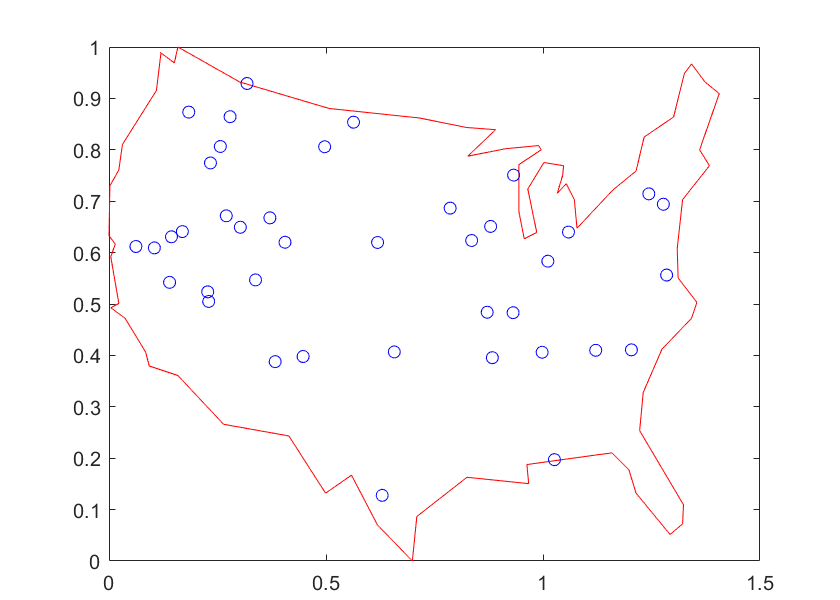• 计算城市间的距离

distances = zeros(cities);

for count1=1:cities
for count2=1:count1
x1 = locations(count1,1);
y1 = locations(count1,2);
x2 = locations(count2,1);
y2 = locations(count2,2);
distances(count1,count2)=sqrt((x1-x2)^2+(y1-y2)^2);
distances(count2,count1)=distances(count1,count2);
end
end

• 定义目标函数

FitnessFcn = @(x) traveling_salesman_fitness(x,distances);

my_plot = @(options,state,flag) traveling_salesman_plot(options,
state,flag,locations);

• 设置优化属性并执行遗传算法求解

options = optimoptions(@ga, ’PopulationType’‘custom’,‘InitialPopulationRange’
[1;cities]);

options = optimoptions(options,‘CreationFcn’,@create_permutations,
‘CrossoverFcn’,@crossover_permutation,
‘MutationFcn’,@mutate_permutation,
‘PlotFcn’, my_plot,
‘MaxGenerations’,500,‘PopulationSize’,60,
‘MaxStallGenerations’,200,‘UseVectorized’,true);

numberOfVariables = cities;
[x,fval,reason,output] =
ga(FitnessFcn,numberOfVariables,[ ],[ ],[ ],[ ],[ ],[ ],[ ],options);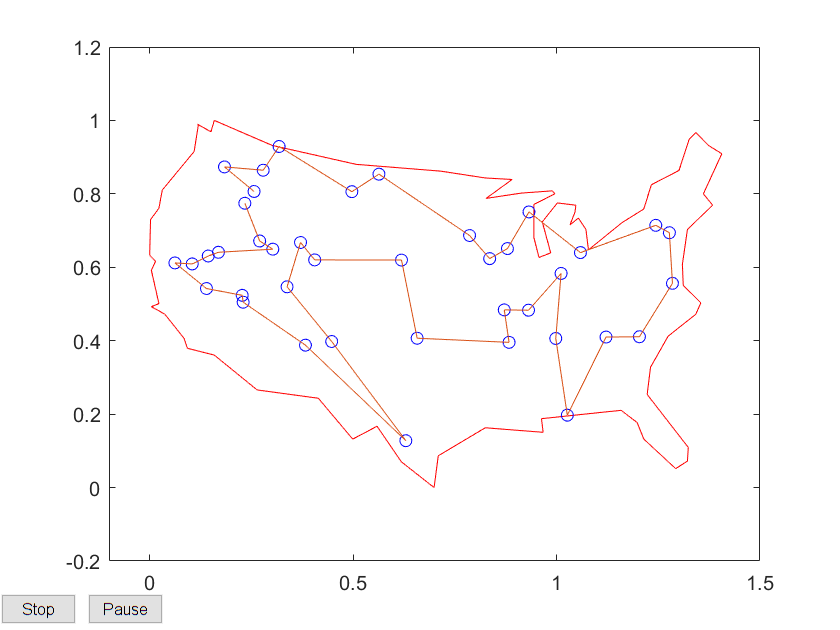3. 模拟退火算法

• 用模拟退火(SA)算法求解Peaks问题

clc, clear, close all

• 定义优化问题

peaks

problem = createOptimProblem(’fmincon’,
‘objective’,@(x) peaks(x(1),x(2)),
‘nonlcon’,@circularConstraint,
‘x0’,[-1 -1],
‘lb’,[-3 -3],
‘ub’,[3 3],
‘options’,optimset(‘OutputFcn’,
@peaksPlotIterates))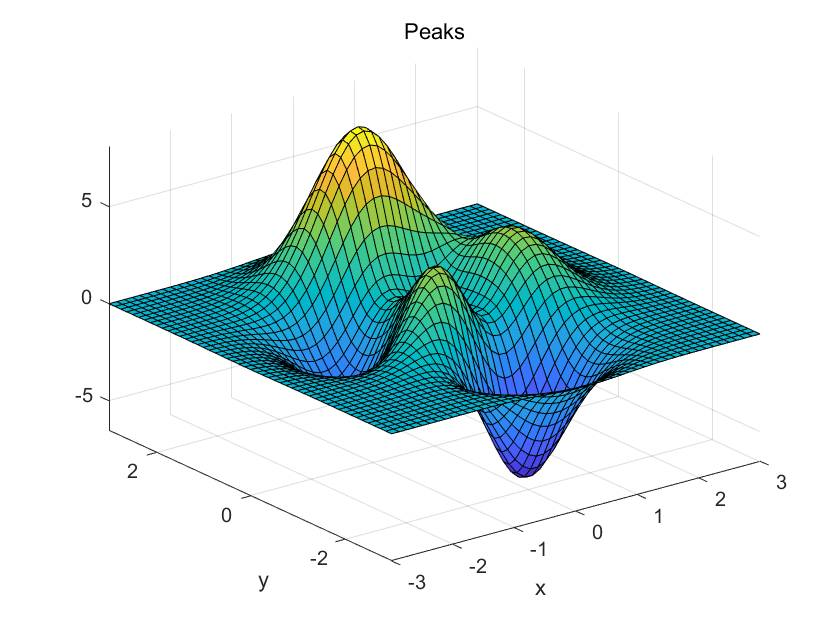[x,f] = fmincon(problem)

x =

-1.3474    0.2045
f =
-3.0498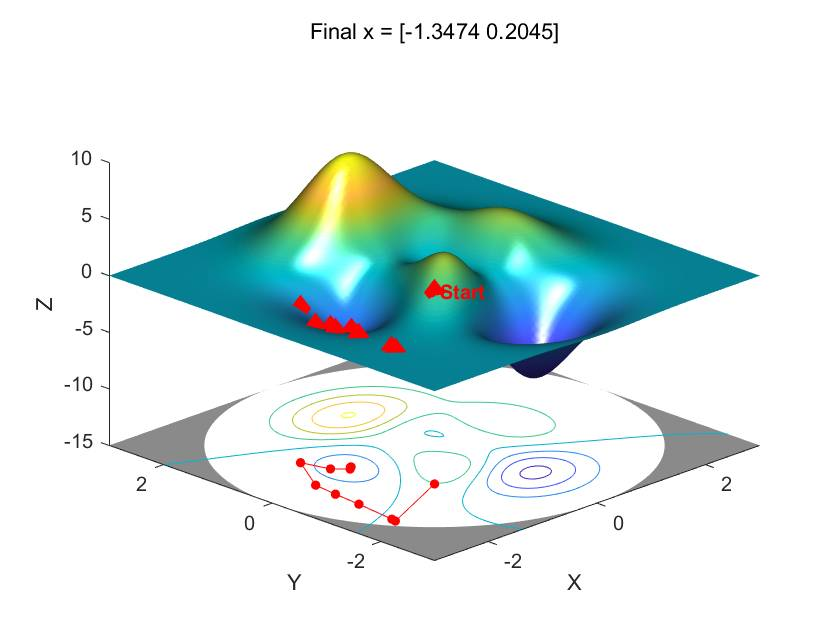• 用 SA 算法寻找全局最小值

problem.solver  = ’simulannealbnd’;

problem.objective = @(x) peaks(x(1),x(2)) + (x(1)^2 + x(2)^2 - 9);
problem.options = saoptimset(’OutputFcn’,@peaksPlotIterates,
‘Display’,‘iter’,
‘InitialTemperature’,10,
‘MaxIter’,300)

[x,f] = simulannealbnd(problem)

x =
0.2280   -1.5229
f =
-13.0244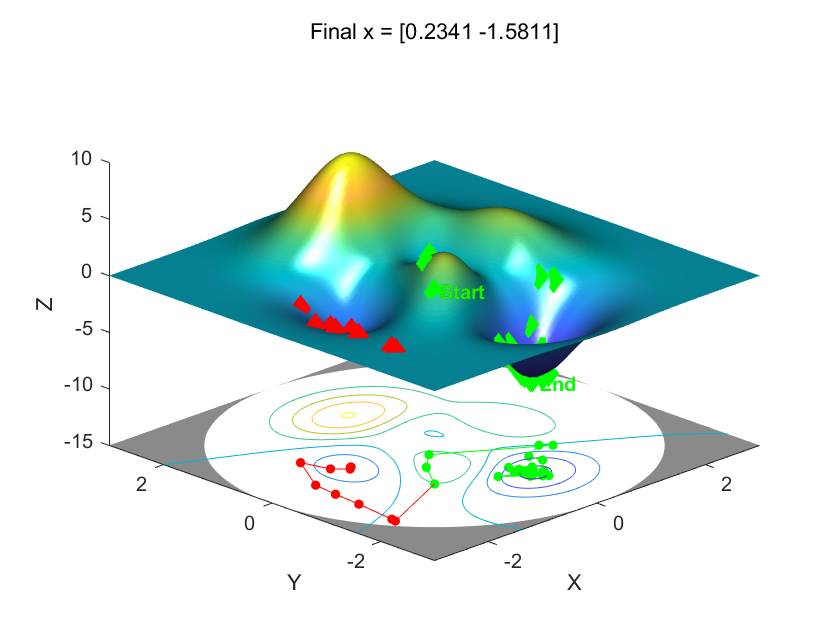4. 全局优化求解器汇总

MATLAB 全局优化算法的各求解器如下表所示。 在建模比赛中， 建议大家先了解各算法的原理， 这样当遇到具体问题的时候， 就可以根据问题的特征判断哪个或哪几个算法比较合适， 如果不好判断， 不妨全部尝试一下， 做个算法比较也是比较常见的事情， 这样得到的结果更酷， 摘要也更有内容啦。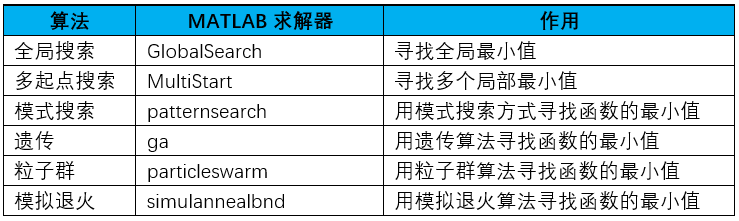©️2019 CSDN 皮肤主题: 大白 设计师: CSDN官方博客